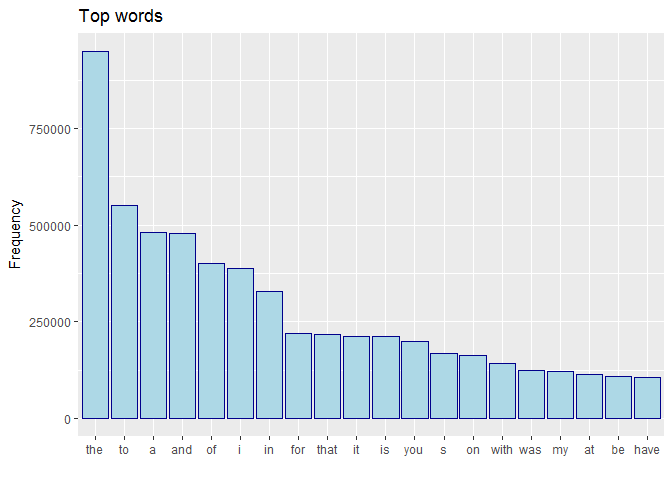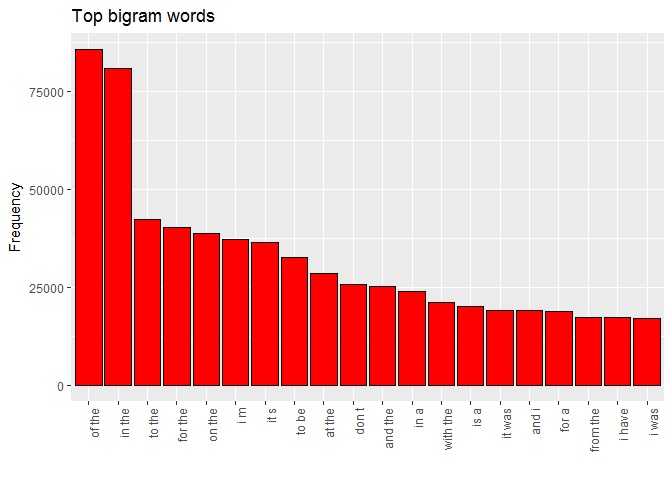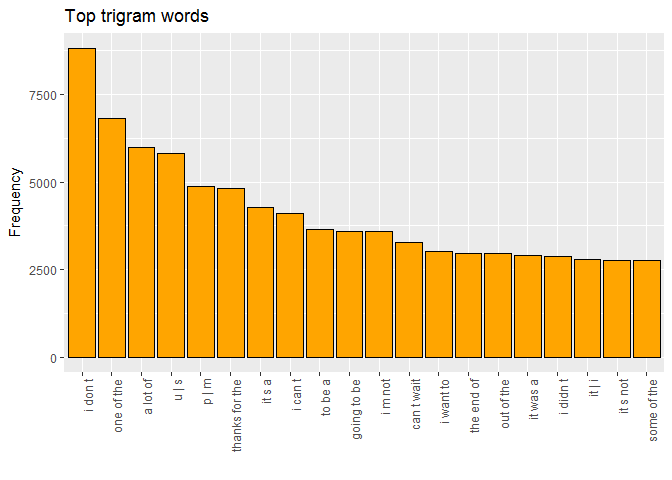# Data Science Specialization Capstone Project: Milestone Report

This is my Milestone Report for the Data Science Capstone Project, last step of the  Data Science Specialization  by Johns Hopkins University on Coursera.
You can see the Md, Rmd and html format here, the compiled html result here.
The goal of the Capstone Project was to implement a useful model for predict a word given one or more words and to develop a web app to use it. In the Milestone Report I have to clean and explore the data.

------------------------------------------------------------------------

## Summary

In this report there are the steps for cleaning and for the main exploratory data analysis of the datasets. The steps are:
1. Import the data
2. Main characteristics of the datasets
3. Merge and sample the datasets
4. Clean the dataset
5. Word frequencies
6. Bigram frequencies
7. Trigram frequencies
8. Next steps

## 1. Import the Data

``````# twitter

# news
conn <- file("Capstone Project/Coursera-SwiftKey/final/en_US/en_US.news.txt", open="rb")
close(conn)
rm(conn)

# blogs
blogs <- iconv(blogs, from = "latin1", to = "UTF-8", sub="")``````

## 2. Main characteristics of the datasets

For each dataset we see number of lines and words.
``````library(stringi)

# Lines and words
paste('We have ',length(twitter)," tweets with a total of ",stri_stats_latex(twitter), " words.", sep = "")``````
``##  "We have 2360148 tweets with a total of 30553755 words."``
``paste('We have ',length(news)," news articles with a total of ",stri_stats_latex(news), " words.", sep = "")``
``##  "We have 1010242 news articles with a total of 34494539 words."``
``paste('We have ',length(blogs)," blog posts with a total of ",stri_stats_latex(blogs), " words.", sep = "")``
``##  "We have 899288 blog posts with a total of 37864968 words."``

## 3. Merge and sample the datasets

I merge the three datasets in a single one.
I tried to use all the data but it was not possible because of the limited memory of my notebook. For this reason I take 20 percent of all datasets. We will have over 10 million words anyway.
``````DataComplete <- c(twitter,blogs,news)
set.seed(190587)
DataSample <- sample(DataComplete, length(DataComplete)*0.2)
paste('We have a total of ',length(DataSample)," sentences with ",stri_stats_latex(DataSample), " words.", sep = "")``````
``##  "We have a total of 853935 sentences with 20581030 words."``

## 4. Clean the dataset

First I delete some symbols: for examples, Twitter user use the # for the topics, then I must replace it.
Other symbols like punctuation (except comma) are used for terminate a sentence and start a new one: when there is these symbols I restart the string in a new line.
I also convert all the characters to lower, and delete multiple spaces.
``````# clean the Data
DataClean <- gsub("#"," ", DataSample)
DataClean <- gsub("\'"," ", DataClean)
DataClean <- gsub("\""," ", DataClean)
DataClean <- gsub("-"," ", DataClean)
DataClean <- gsub("_"," ", DataClean)

# split each line When there is a punctuation (except for comma).
DataClean <- unlist(strsplit(DataClean, ".", fixed=TRUE))
DataClean <- unlist(strsplit(DataClean, "!", fixed=TRUE))
DataClean <- unlist(strsplit(DataClean, "?", fixed=TRUE))
DataClean <- unlist(strsplit(DataClean, ":", fixed=TRUE))
DataClean <- unlist(strsplit(DataClean, ";", fixed=TRUE))
DataClean <- unlist(strsplit(DataClean, "(", fixed=TRUE))
DataClean <- unlist(strsplit(DataClean, ")", fixed=TRUE))
DataClean <- unlist(strsplit(DataClean, "/", fixed=TRUE))

# insert symbol | after each sentence
DataClean <- paste(DataClean,"|")

# convert in lower all the letter
DataClean <- tolower(DataClean)

# delete white spaces before and after any sentence
DataClean <- trimws(DataClean)

# convert double spaces or more in a single one
DataClean <- gsub("\\s+", " ", DataClean)``````
Now I split each word within a sentence.
``````# divide the single words
DataClean <- unlist(strsplit(DataClean, "\\s"))``````

## 5. Word frequencies

We split the dataset for every word then we find the most frequent words and see these through an histogram.
``````library(ggplot2)

# convert in data.frame
DFWord <- data.frame(unlist(DataClean))

# find frequencies and order
DataFreq <- as.data.frame(table(DFWord))
DataFreq <- DataFreq[order(-DataFreq\$Freq),]

# delete "|" (unnecessary for frequencies)
DataFreq <- DataFreq[!(DataFreq\$DFWord=="|"),]

# take top 20 for the graph and order
DataTop\$DFWord <- factor(DataTop\$DFWord, levels = DataTop\$DFWord[order(-DataTop\$Freq)])

# graph
g <- ggplot(DataTop, aes(DataTop\$DFWord,DataTop\$Freq))
g + geom_bar(stat='identity', col="dark blue", fill="light blue")+labs(title="Top words", x="",y="Frequency")``````## 6. Bigram frequencies

We want the most frequent two consecutive words. For do this I have to take all the two consecutive words in each sentence, then we have to replace all the couples with a “|”.
``````#Load libraries
library(quanteda)

# Two consecutive words
Data2Gram <- tokens_ngrams(DataClean, concatenator=" ")

# Delete couples with "|"
Data2Gram <- grep("^[^\\|]", Data2Gram, perl=TRUE, value=TRUE)
Data2Gram <- grep("[^\\|]\$", Data2Gram, perl=TRUE, value=TRUE)``````
Now we calculate all the frequencies and see the top 20 through an histogram.
``````# convert in data.frame
DF2gram <- data.frame(unlist(Data2Gram))

# find frequencies and order
Freq2Gram <- as.data.frame(table(DF2gram))
Freq2Gram <- Freq2Gram[order(-Freq2Gram\$Freq),]

# take top 20 for the graph and order

# graph
gg + geom_bar(stat='identity', col="black", fill="red")+labs(title="Top bigram words", x="",y="Frequency")+theme(axis.text.x = element_text(angle = 90, hjust = 1))``````## 7. Trigram frequencies

We want now the most frequent three consecutive words.
``````# Two consecutive words
Data3Gram <- tokens_ngrams(DataClean, n = 3L, concatenator=" ")

# Delete couples with "|"
Data3Gram <- grep("^[^\\|]", Data3Gram, perl=TRUE, value=TRUE)
Data3Gram <- grep("[^\\|]\$", Data3Gram, perl=TRUE, value=TRUE)``````
Now we calculate all the frequencies and see the top 20 through an histogram.
``````# convert in data.frame
DF3gram <- data.frame(unlist(Data3Gram))

# find frequencies and order
Freq3Gram <- as.data.frame(table(DF3gram))
Freq3Gram <- Freq3Gram[order(-Freq3Gram\$Freq),]

# take top 20 for the graph and order

# graph
gg + geom_bar(stat='identity', col="black", fill="orange")+labs(title="Top trigram words", x="",y="Frequency")+theme(axis.text.x = element_text(angle = 90, hjust = 1))``````## 8. Next steps

In this report we have a first look at the data.
The next steps will be:
1) Create the prediction algorithm: in particular the bigram and the threegram model will be used for the prediction algorithm.
2) Build the app: giving one or more words, the prediction app will be suggest a list of possibles “next word” using the prediction algorithm.